Next: Spherical symmetry Up: The Skyrme Hartree-Fock-Bogolyubov equations Previous: The Skyrme Hartree-Fock-Bogolyubov equations

## Local densities

In the Skyrme-HFB formalism, the evaluation of the expectation value of the energy leads to an expression which is a functional of the local densities, namely, the particle (normal) and pairing (abnormal) densities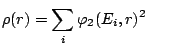and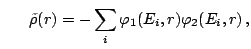(4)

and their derivatives. The presence of non local terms in the force leads to a dependence on the normal and abnormal kinetic densities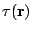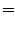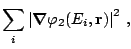(5)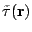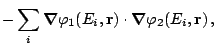(6)

while the spin-orbit term leads to a dependence on the spin current tensors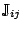and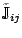. We do not give here the definitions of these tensors, because for the spherical symmetry discussed here they reduce to the corresponding spin current vectors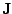and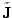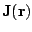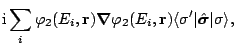(7)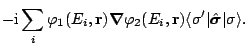(8)Next: Spherical symmetry Up: The Skyrme Hartree-Fock-Bogolyubov equations Previous: The Skyrme Hartree-Fock-Bogolyubov equations
Jacek Dobaczewski 2005-01-23